# Terahertz Communication with MIMO-OFDM in FANETs for 6G

Nazifa Mustari1, Muhammet Ali Karabulut2, A. F. M. Shahen Shah1, *, Ufuk Tureli1
1 Department of Electronics and Communication Engineering, Yildiz Technical University, Istanbul, Turkiye
2 Department of Electrical and Electronics Engineering, Kafkas University, Kars, Turkiye

#### Article Metrics

0
##### Total Statistics:

Full-Text HTML Views: 297
Abstract HTML Views: 111
##### Unique Statistics:

Full-Text HTML Views: 170
Abstract HTML Views: 87open-access license: This is an open access article distributed under the terms of the Creative Commons Attribution 4.0 International Public License (CC-BY 4.0), a copy of which is available at: https://creativecommons.org/licenses/by/4.0/legalcode. This license permits unrestricted use, distribution, and reproduction in any medium, provided the original author and source are credited.

* Address correspondence to this author at the Department of Electronics and Communication Engineering, Yildiz Technical University, Istanbul, Turkiye; E-mail: shahen.shah@hotmail.com

## Abstract

### Background:

Unmanned aerial vehicles (UAVs) are envisioned in several intelligent transport system (ITS) application fields due to their autonomous operation, mobility, and communication/processing capabilities.

### Methods:

In order to increase performance, dependability, and customer satisfaction, wireless communications are being used for essential and noncritical services in a variety of transportation systems. Terahertz (THz) communication aims to raise the wireless communication frequency band to the terahertz level and is one of the technologies that shows the most promise in addressing needs like the exponential increase in the number of devices, the necessity for higher data rates, and the need for larger channel capacity. For meeting the demands of 5G and 6G users, the hybrid combination of multiple input multiple output (MIMO) and orthogonal frequency division multiplexing (OFDM) appears to be an excellent technology. Therefore, it is essential to investigate the performance of THz communication with MIMO-OFDM in flying ad hoc networks (FANETs) for 6G. FANETs are multi-UAV systems arranged in an ad hoc way.

### Results:

In this paper, the performance of the THz communication with MIMO-OFDM in FANETs for 6G is evaluated. The Markov chain model-based analytical study is used to derive how parameters and performance measures relate to one another.

### Conclusion:

The equations for path loss, outage probability, bit error rate, and throughput are attained. Moreover, numerical results are shown to justify the outcomes of the analytical studies.

Keywords: FANETs, MIMO, OFDM, Terahertz, UAVs, 6G.

## 1. INTRODUCTION

One of the most promising new technologies for intelligent transport system (ITS) infrastructure is unmanned aerial vehicles (UAVs) . In contrast to manned vehicle operations, UAVs, a.k.a. drones or remotely piloted aircraft, protect humans. Aerial photography, search and rescue missions, radar localization, agricultural monitoring, observation support, border surveillance, weather, and environmental monitoring, and other activities are only a few of the many uses for UAVs in both civil and military operations [1, 2]. Over the next decade, UAVs are predicted to be used in a growing number of contexts, and this growth will necessitate the support of more robust wireless networks beyond 5G and 6G . Both UAV-to-UAV (U2U) and UAV-to-Infrastructure (U2I) communications are supported by UAV ad hoc networks, which are another name for flying ad hoc networks (FANETs).

From 0.1 to 10 THz is the frequency range of the THz band . In Fig. (1), the spectrum is presented. THz frequency spectrum is seen as a possible contender to enable ultra-broadband for the future beyond the fifth generation (5G), filling the gap between millimeter wave (mmWave) and optical frequency ranges in light of the exponential rise of data traffic in wireless communication systems . The THz range has shown promise as a channel for high-rate data transport  and provides benefits, including smaller size and wider bandwidth . However, it has drawbacks, including spreading loss and absorption loss that are brought on by high-frequency features. It is crucial to use diversity methods, such as multiple-input multiple-output (MIMO) , to combat these impacts.

High spectrally efficient wireless transmissions have shown considerable potential using MIMO technology at the physical layer. Orthogonal frequency division multiplexing (OFDM) has become the PHY layer technology. High data transmission rates may thus be achieved by combining MIMO with OFDM . MIMO uses many antennas simultaneously and may increase data throughput via multipath propagation and random fading. Theoretically, the MIMO system, which has multiple antennas in both the transmitter and the receiver, provides a linear link capacity increase. The rank of the MIMO channel determines the capacity. While spatial multiplexing may achieve excellent spectrum performance, several alternative MIMO systems, including those that use spatial diversity, spatial-temporal coding, and interface cancellation, can enhance signal quality and coverage . The use of OFDM as a multi-carrier modulation technique for wireless signal transmission has become increasingly popular. Multi-symbol, multi-rate, and multi-carrier transmission principles are among them. Since OFDM reduces inter-symbol interference, subcarrier overlap is possible without inter-carrier interference. These methods used together will enable devices to achieve line of sight (LoS) criteria, including strong coverage, high peak data rates, effective transmission, and great spectral performance. Multiple antennas in transmitter and receiver considerably increase channel capacity and signal-to-noise ratio (SNR) in order to satisfy the need for rapid data transfers [6, 7]. Besides, the outstanding spectral quality of OFDM makes it a popular choice for signal transmission in systems for wireless communication. In next-generation communication systems, MIMO-OFDM, which combines MIMO and OFDM technologies to achieve great spectral efficiency, is crucial.

Great data speeds and high spectral efficiency are provided by MIMO methods, which use several antennas on both sides of a wireless connection [8, 9]. Research on MIMO systems has mostly concentrated on narrowband channels. On the other hand, MIMO channels provide high utilization of capacity, frequency variety, and spatial diversity. Since OFDM  greatly decreases receiver complexity, MIMO technology with OFDM  is an interesting approach for next-generation broadband wireless communication networks. Intercarrier interference and multipath fading greatly lower MIMO-OFDM system performance [12, 13]. In recent years, MIMO THz communication has attracted a lot of attention. The authors [14, 15] explored indoor short-range MIMO THz communications considering the significant signal attenuation. Despite a few contributions on OFDM for UAV systems [16, 17], these methods relied on the specifically planned simplicity of traditional micro/millimeter channels and are still not suitable for THz communications. Although authors of [18, 19] researched UAVs, THz path loss for UAVs was not taken into account.

Following is a summary of the study's contributions. The effectiveness of THz communication using MIMO-OFDM in FANETs for 6G is assessed in this study. We present a Markov-chain mathematical model for modeling the system. The relationship between parameters and performance metrics is deduced. We derive the expressions for bit error rate (BER), throughput, outage probability, and path loss. Furthermore, numerical results are shown to support the conclusions of the analytical studies.

The rest of this work is structured as follows. THz communication with the MIMO-OFDM system is described in Section 2. Performance analysis is provided in Section 3. Section 4 reviews the simulation results, while Section 5 concludes the manuscript.

## 2. METHODS

### 2.1. The Communication with MIMO-OFDM

Data rates and coverage for connecting FANETs to current cellular networks are expected to increase by 5G technology . Additionally, 5G offers multi-access edge computing with cloud computing features. High-altitude UAVs with GPS, sensors and cameras may benefit greatly from 5G. Additionally, 6G has the capacity to include autonomous services and yet-to-be-envisioned new advancements, making it convenient to conceive cellular networks that go beyond 5G . The two key concerns are ensuring privacy for tiny UAVs in next-generation wireless networks and the safe use of these technologies . In order to greatly stabilize battery life, have low computational costs, and promote improved communication, it is crucial to have suitable wireless technologies.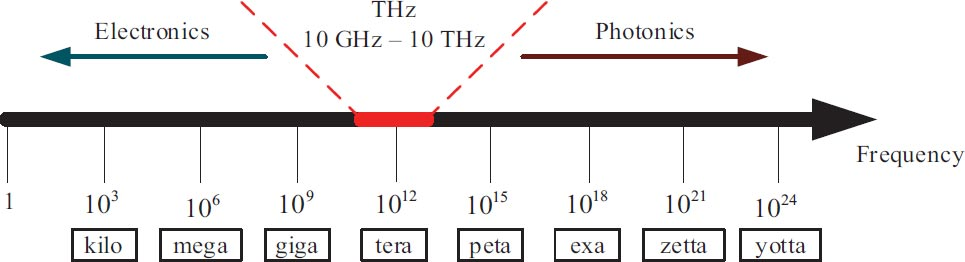Fig. (1). THz frequency spectrum.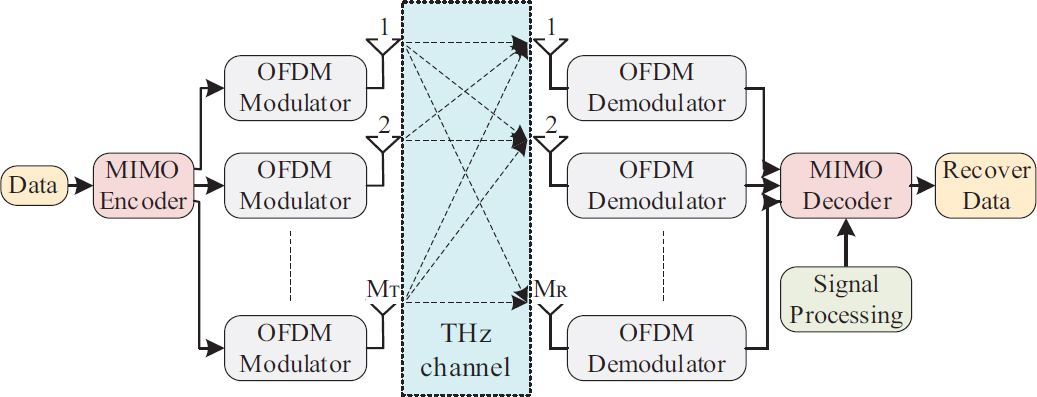Fig. (2). MIMO-OFDM system over THz communication channel.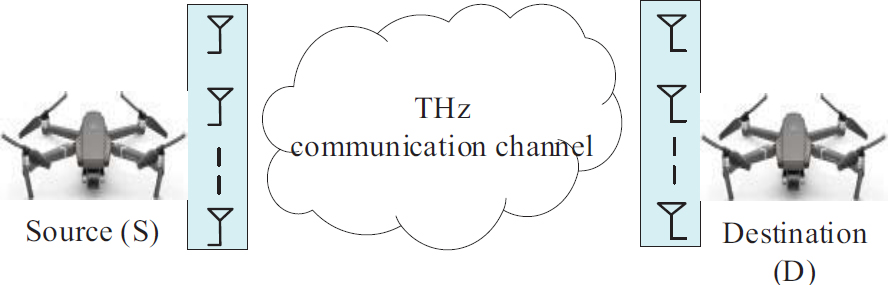Fig. (3). MIMO scenario.

The peak data rate required by 6G networks is projected to reach one terabit per second (Tb/s) which is 100 times faster than that of 5G [23, 24]. This is way faster than what the most advanced wireless systems can now provide. The great potential provided by the massively accessible bandwidths of the THz band must be investigated in order to satisfy this need. The whole THz band is split into ultra-wideband transmission windows and absorption coefficient peak areas due to the frequency-dependent nature of absorption loss. Due to its adaptability in combining with various technologies, OFDM has been recommended as the modulation waveform for 6G networks [25-27]. In OFDM, data is delivered via overlapping spectra from a number of closely spaced orthogonal subcarrier signals. MIMO-OFDM is now regarded as one of the most cutting-edge technologies for next-generation cellular wireless networks. Combining MIMO and OFDM systems has garnered a lot of attention due to the rising need for multimedia applications. Two techniques for enhancing wireless communication networks are diversity and spatial multiplexing. The resilience of the communication equipment in regard to BER is increased by having several pathways between the antennas. Fig. (2) depicts a MIMO-OFDM system over the THz communication channel.

In , a performance evaluation of using the THz band in FANETs is made. However, the effect of the MIMO-OFDM system on performance was not examined. In this study , the performance of MIMO-OFDM systems at THz frequencies was investigated. Simulations using different antenna structures and various channel models showed that the THz frequency band is suitable for high-speed data transfer. However, the effect of FANET structures on 6G was not examined. This study  proposed a combination of MIMO-OFDM techniques using the THz frequency band in 6G networks. It is thought to be suitable for high-speed data transfer. However, FANET structures were not studied. Our study provides essential information about the use of MIMO-OFDM and THz communication in FANETs.

## 3. PERFORMANCE ANALYSIS

As shown in Fig. (3), we examine a FANET scenario in which each UAV node is equipped with several antennas for THz communication. Each UAV has MT and MR number of antennas for the transmitter and receiver sides, respectively. Different studies have proposed various approaches to estimate the path loss across a channel at THz frequencies. The amount of path loss experienced by a transmission from its source (S) to its final destination (D) varies with both frequency and physical distance, which can be given as :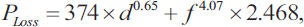(1)

where the distance between the two UAV nodes, denoted by d, is the transmission distance. At distance d, the signal power Pr(d) can be given as :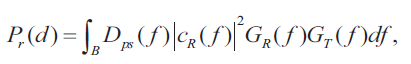(2)

where the transmitted signal's power spectral density is denoted by Dps. The gain of the transmitter, indicated by GT, and the gain of the receiver, denoted by GR. cR, is the Terahertz channel frequency response. Dps can be given as: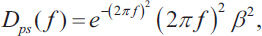(3)

where β denotes a normalizing constant is used to modify the total energy of the pulse and f indicates the frequency of the signal in THz, and bandwidth is expressed as B. cR can be given as: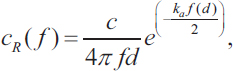(4)

where c denotes light speed, and ka is the medium's molecular absorption coefficient, which is defined by the transmission medium's molecular composition or the kinds and numbers of molecules present in the channel.

The absorption noise in the Terahertz channel is non-white and has a significant degree of frequency selectivity . Thus, the overall bandwidth may be split into several small sub-bands, as well as the capacity can be obtained by adding the capacities of each. Following that, the capacity in bits/s can be given as: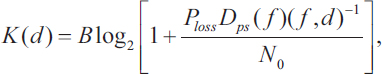(5)

where No is the noise.

The wireless local area network (WLAN) is a packet-switched system with a random access protocol. This effectively implies that a priori is unaware of the timings at which packets will arrive. Due to unpredictable arrival times and high data speeds, synchronization must be finished quickly when a packet begins to be received. The data packet is headed with a recognized sequence (the preamble) to aid “quick” synchronization. Preamble information is crafted to enable accurate packet identification, determination of frequency offset, timing of symbols, and channel estimation. The MIMO system can distinguish between the components of the signal coming from the various transmit antennas when the MIMO channel elements are accurately known.

The ability to identify each subchannel from each transmitting (TX) antenna to each receiving (RX) antenna is crucial for estimating the MIMO channel. To accomplish that, at least for the channel length, the preambles on various TX antennas should be orthogonal and shift-orthogonal. According to Fig. (4), we have selected time orthogonality. Furthermore, a cyclic prefix of length 2Ng is introduced to lessen the vulnerability of the channel estimate to ISI. For a 2x2 system, this results in the preamble as shown in Fig. (4). The training sequence is selected to follow the IEEE 802.11 standard's lengthy training symbol, with Ng=16 and Nc=64, resulting in a nonzero fraction of Ntrain=2(Ng + Nc) samples for each TX antenna.

In a MIMO-OFDM system, each part of the TX antenna to the RX antenna is considered a finite impulse response (FIR) filter in which Rayleigh fading coefficient is gi,j(k) where k=0, ..., M-1, and M represents the number of multi-path. In the nth frequency subcarrier, the N (M<N) point DFT can be given as :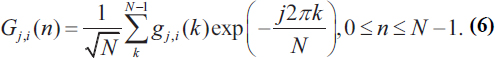(6)

The SNR of the MIMO-OFDM structure in the nth subcarrier, when all antennas are non-correlated, can be given via maximum ratio combining (MRC) diversity :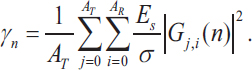(7)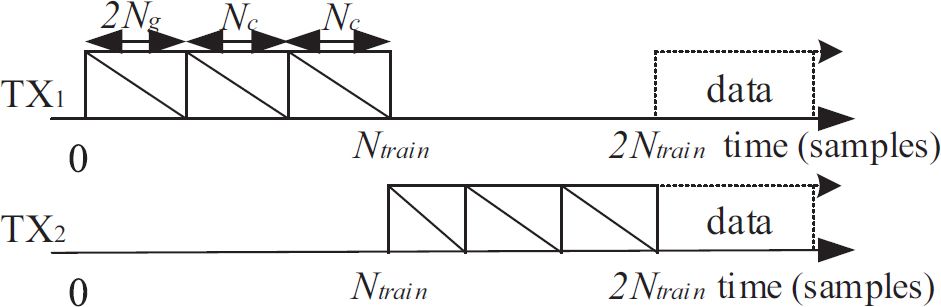Fig. (4). Design of a time-orthogonal preamble for a MIMO configuration using two transmit antennas.

If all receiver antennas are non-correlated, moment generating function can be written as :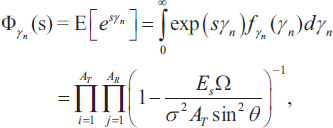(8)

where ƒγn(γn) is the pdf of γn SNR γn denotes the output of the MRC combiner. Ω=E[γn2]. E[.] denotes the expectation operator.

The BER probability for BPSK under a particular value of SNR γn can be obtained by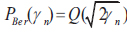Q(.) indicates the Gaussian Q function and can be defined by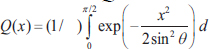It is assumed that number of transmit antennas is equal to the number of receiver antennas (A=AT=AR). For the nth frequency subcarrier, the average BER can be given as :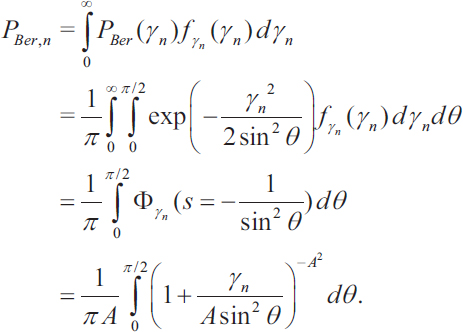(9)

Due to the fact that the frequency subcarrier is no longer a function, the overall average BER for an OFDM system with multiple receiver antennas may be computed as: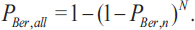(10)

Poutage is the outage probability which is the possibility that the SNR of the received signal R is less than the SNR threshold γth, which can be written as: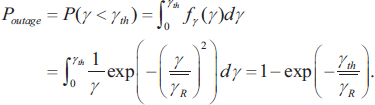(11)

Here, γR denotes the average SNR of the received signal, which can be given as: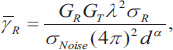(12)

where σR and σNoise denote the power of transmission at the receiver and background noise, respectively. GR = GT = 4π/λ2, and λ = c/f where λ indicates signal wavelength. α symbolizes the path loss exponent.

The Markov chain model developed by Bianchi  is considered in this study. Bianchi's analysis is provided for the IEEE 802.11 DCF scheme, in which the discrete-time Markov chain model is used to calculate each UAV transmission probability (τ) as a function of the conditional collision probability (fPC). The transmission rates of concurrent transmissions are calculated under the assumption that the fPC collision probability is constant across the entire UAV.

A UAV detects the channel in one of three states during a randomly selected time slot: idle (no transmission activity), busy during successful transmission, or busy due to collision. Assuming that a medium is attempting to transmit a frame with probability in an arbitrarily chosen time slot; the expression can be given as :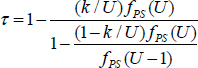(13)

Collision probability fPC can be calculated if a collision takes places when at least one of the U−1 UAVs initiates transmission. Therefore, if 1−τ is exactly the probability that a UAV is idle, (1−τ)U−1 is the probability that the UAV is idle. The probability of collision of at least one of the U-1 UAVs in the transit state can be calculated as: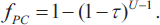(14)

Here ƒPSC is successful transmission probability. ƒPSC can be given as: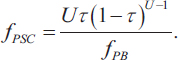(15)

In a successful transmission, there are exactly A number of antennas, so fPS(U) can be obtained as the possibility that only one UAV node wins the transmission opportunity at the end of each contention period. Similarly, fPS can be calculated from the fact that a transmission is successful if only one UAV transmits data, given that at least one UAV in the network transmits among U UAVs :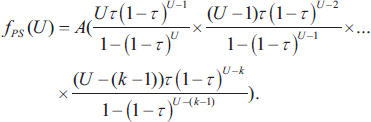(16)

For the first expression, the 1−(1−τ)U denominator denotes the probability of at least one of U UAVs transmitting in a one-time slot, while the Uτ(1− τ)U−1 numerator is exactly U UAVs transmitting in a one-time slot. Thus, given a time slot in which at least one UAV gains contention, the first term represents the probability that exactly one UAV will transmit during that time slot. Except that the previous contentions are calculated in the collision-free state, all remaining A-1 terms can be described in the same way.

The system throughput S can be calculated as the ratio of the average load information transmitted in a time slot to the average duration of a time slot: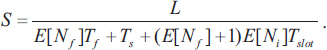(17)

Here, L is the length of transmitted data. Uf denotes the number of failed transmissions during the transmission time, and Ui represents the number of idle time intervals between two consecutive transmissions. Tf and Tslot denote the duration of a failed transmission and the duration of a slot, respectively. Ts and Tf can be given as: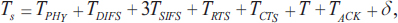(18)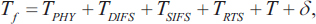(19)

where TDIFS, TSIFS, TRTS, TCTS, TACK and, δ DCF indicate interframe gap time, short interframe gap time, RTS time, CTS time, transmission time of ACK frame and propagation delay. Their values are listed in Table 1. The expression for the expected values of Uf and Ni can be calculated as follows: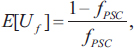(20)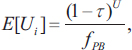(21)

where fPB indicates the probability of at least one transmission in the considered time slot and fPS is the probability of successful transmission. fPB is calculated as: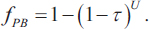(22)

## 4. NUMERICAL RESULTS & DISCUSSION

This section evaluates the performance of a THz communication with MIMO-OFDM in FANETs for 6G. MATLAB program is used to obtain numerical results. The values of parameters for the numerical results are given in Table 1.

Fig. (5) shows path loss against THz frequency. With an increase in terahertz frequency, path loss increases. Thus, it may be concluded that lower frequencies in the THz band will likely result in links of higher quality. Moreover, path loss becomes worse as you go further. Because radio waves spread out according to the inverse square law and diminish with the square of the wavelength of the radio waves, path loss rises with the square of the distance between the receiver and transmitter antennas.

Table 1. The utilized values of parameters in numerical results.
Parameters Values
Tslot, TSIFS, TdelayS) 30, 10, 50, 3
CW, mr 64, 5
RTS, CTS, ACK,Lh, L (bytes) 26, 20, 14, 50, 512
Rd (Mbps), Rc (Mbps), B (Gbps) 11, 1, 100
U, Usc 150, 48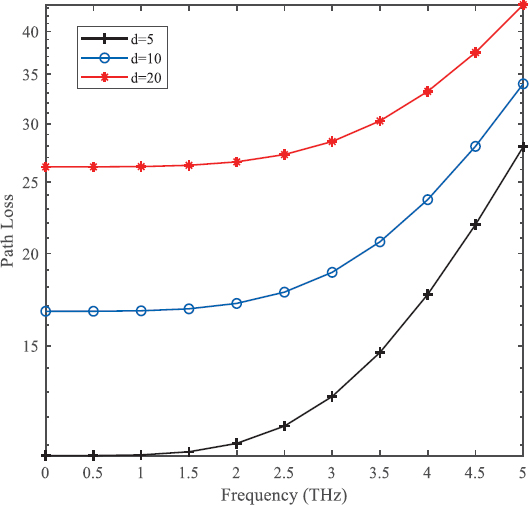Fig. (5). Path loss versus frequency with different distance.

Fig. (6) presents outage probability vs SNR. According to Fig. (6), the probability of an outage decreases as the SNR threshold value increases because signal strength increases with SNR. The outage probability falls as the number of antennas increases. With more antennae, the probability of an outage decreases even more. This is because the MRC combining at the receiver may have more branches and greater selection diversity as there are more antennas.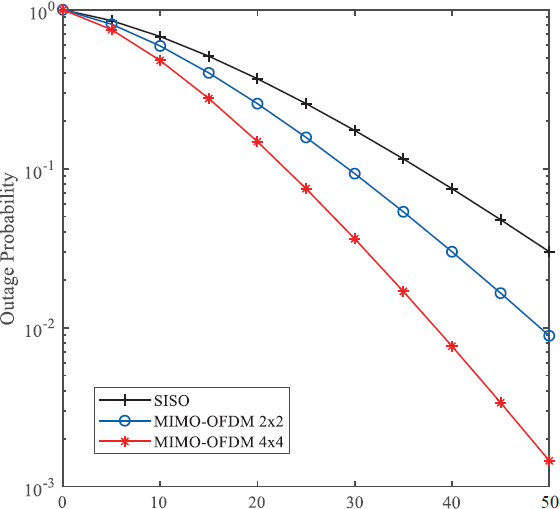Fig. (6). Outage probability against SNR.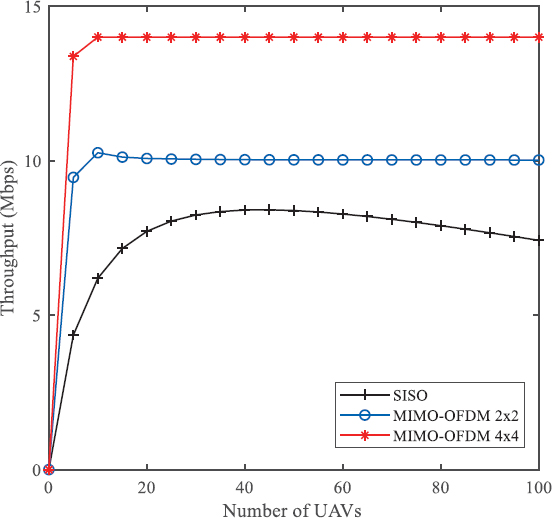Fig. (7). Throughput vs number of UAVs.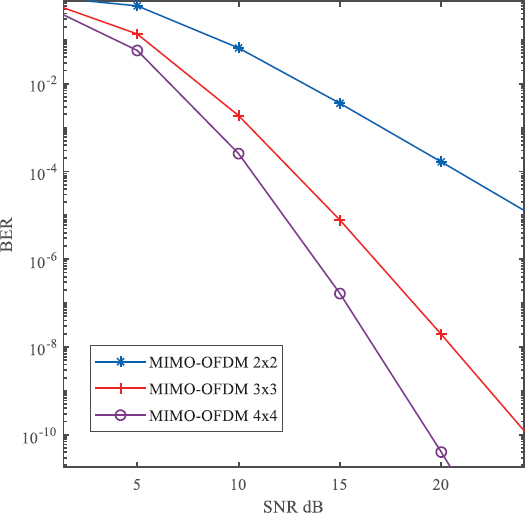Fig. (8). BER versus SNR.

Fig. (7) presents the throughput for the different numbers of UAVs. As the number of UAVs increases up to a certain limit, the data rate increases. Then the data rate starts to decrease significantly because, after this limit, there will be increased contention and more collisions due to excess packets in the channel for transmission. In addition, the data rate is higher in single input single output (SISO) systems up to a certain number of UAVs. This is because the delay is less. However, when the number of UAVs in the network exceeds a certain number, an increase in performance due to MIMO is observed. The performance improves as the number of antennas in the system increases. It can be used to improve performance since an addition in the number of antennas means an efficient increase in its diversity.

Fig. (8) presents the average BER vs SNR. System performance rises with increasing SNR. The increasing number of antennas decreases BER, i.e., increases performance. When the number of antennas extends, the accuracy of signal estimation gets better.

## CONCLUSION

The effectiveness of THz communication using MIMO-OFDM in FANETs for 6G is assessed in this article. The analytical study based on the Markov chain is provided. The relationship between parameters and performance metrics is deduced. Path loss, outage probability, BER, and throughput equations are obtained. Moreover, numerical outputs are shown to support the conclusions of the analytical research. Path loss rises with the increase in the terahertz frequency. The probability of an outage decreases as the SNR threshold value increases. Moreover, system performance increases with increasing SNR, and the increasing number of antennas decreases BER and increases throughput, increasing performance.

## LIST OF ABBREVIATIONS

 UAVs = Unmanned Aerial Vehicles ITS = Intelligent Transport System MIMO = Multiple Input Multiple Output

Not applicable.

## FUNDING

The study is funded by Kafkas University's scientific research project coordination under the 2022-FM-90 project.

### CONFLICT OF INTEREST

The authors declare no conflict of interest, financial or otherwise.

Declared none.